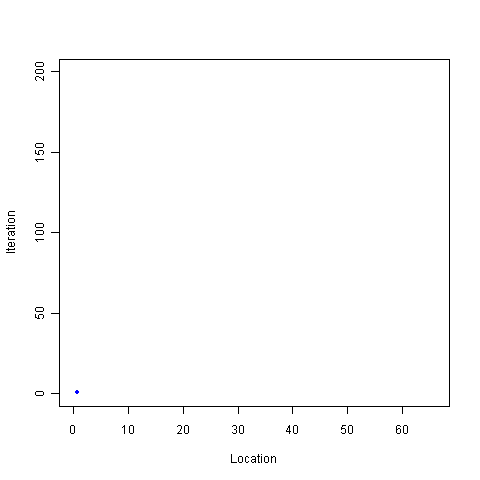## Reaching escape velocitySample once from the Uniform(0,1) distribution. Call the resulting value [latex]x[/latex]. Multiply this result by some constant [latex]c[/latex]. Repeat the process, this time sampling from Uniform(0, [latex]x*c[/latex]). What happens when the multiplier is 2? How big does the multiplier have to be to force divergence. Try it and see:

```iters = 200
locations = rep(0,iters)
top = 1
multiplier = 2
for(i in 1:iters) {
locations[i] = runif(1,0,top)

top = locations[i] * multiplier
}

windows()
plot(locations[1:i],1:i,pch=20,col="blue",xlim=c(0,max(locations)),ylim=c(0,iters),xlab="Location",ylab="Iteration")

# Optional save as movie, not a good idea for more than a few hundred iterations. I warned you!
# library("animation")
# saveMovie(for (i in 1:iters) plot(locations[1:i],1:i,pch=20,col="blue",xlim=c(0,max(locations)),ylim=c(0,iters),xlab="Location",ylab="Iteration"),loop=1,interval=.1)
```

1.Eric

Deriving the distribution of the r.v.s gets messy after t=2 or so, but you can derive the first two moments of this process by inducting over conditional expectations:

E[x(t)]=(1/2)*(c/2)^(t+1)
E[x(t)^2]=(c^(2(t-1)))/(3^t)

so the expectation ->0 if |c|infty if |c|>2, and ->1/2 if c=2.

the second moment ->0 if |c|infty if |c|>3^.5 and ->1/c^2 if |c|=3^.5

Apologies if I made a typo but I think the idea is more or less correct.

2.Eric

So just a comment on your simulation– the expectation should converge to 1/2, but as you can tell, there is some volatility that will become infinitely bad as t->infty.

I think it is possible to derive the mgf of the process in terms of the mgf of the Unif(0,1) which is just 1/(n+1).

3.Matt Asher

I think my questions was too vague.

Take a look at the final value of the sequence for high values of “iters”. See how often you “end up” at a high number, let alone marginally above 0. There is a particular multiplier that seems to guarantee that you will end up with a big number instead of tending to 0.

To make the testing easier I bundled things up in a function that works like a distribution you can sample from:

http://www.statisticsblog.com/code/multiUni.r

Try running this:

z = multiUni(1000,1000,2.1)
mean(z)

That’s an estimator of the expectation after 1000 trials with 1000 iterations each, using multiplier 2.1.

4.John Johnson

While conditional expections is the way to go for deriving a closed expression for expected value, I’m not sure that Eric’s answer is the correct one. The limits of integration in the expectation is a little strange, and I think you end up with an odd product series for the expectation, but I need to check my algebra.